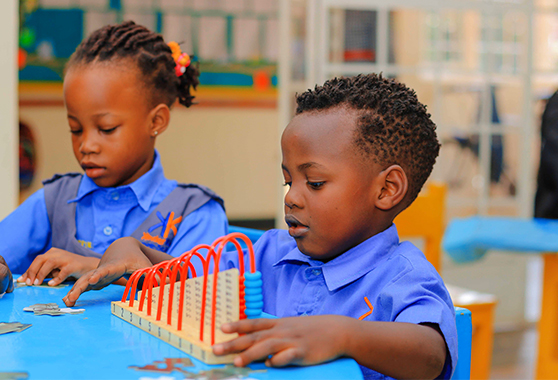admin@Kinderkareschools.com +256 774 209 988 +256 701 533 315

# Mathematics

The Cambridge Primary Mathematics curriculum framework provides a solid foundation for the next stages of education, such as Cambridge Lower Secondary.

The first four content areas are underpinned by Problem solving, which describes the use of techniques, skills and the application of understanding and strategies in solving problems.

Mental strategies are also a key part of the Number content. This curriculum focuses on principles, patterns,
systems, functions and relationships so that learners can apply their mathematical knowledge and develop a holistic understanding of the subject.

There is a curriculum framework for each Cambridge Primary subject. Each framework is organised into six stages. They reflect the teaching target for each year group and provide comprehensive learning objectives. We organise the Cambridge Primary Mathematics curriculum in five content areas or ‘strands’. Each strand is further subdivided into ‘sub-strands’.

#### Numbers

• Numbers and the number system.
• Calculation – Mental strategies, Addition and subtraction, Multiplication and division.

#### Geometry

• Shapes and geometric reasoning.
• Position and movement.

#### Measure

• Money (until Stage 3).
• Length, mass and capacity.
• Time.
• Area and perimeter (from Stage 4).

#### Handling data

• Organising, categorising and representing data.
• Probability (from Stage 5).

#### Problem solving

• Using techniques and skills in solving mathematical problems.
• Using understanding and strategies in solving problems (from Stage 3).Cambridge Primary starts learners on an exciting educational journey, setting out what they should
be able to do at each stage of their primary education.